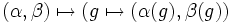# Homomorphism set to direct product is Cartesian product of homomorphism sets

## Statement

### For groups

Suppose$A,B,C$ are groups. Denote by$B \times C$ the external direct product of the groups$B$ and$C$. Denote by$\operatorname{Hom}(A,B)$,$\operatorname{Hom}(A,C)$, and$\operatorname{Hom}(A,B \times C)$ the homomorphism sets between the pairs of groups. There is a canonical bijection:$\operatorname{Hom}(A,B) \times \operatorname{Hom}(A,C) \leftrightarrow \operatorname{Hom}(A,B \times C)$

The bijection is defined as:$(\alpha,\beta) \mapsto (g \mapsto (\alpha(g),\beta(g))$